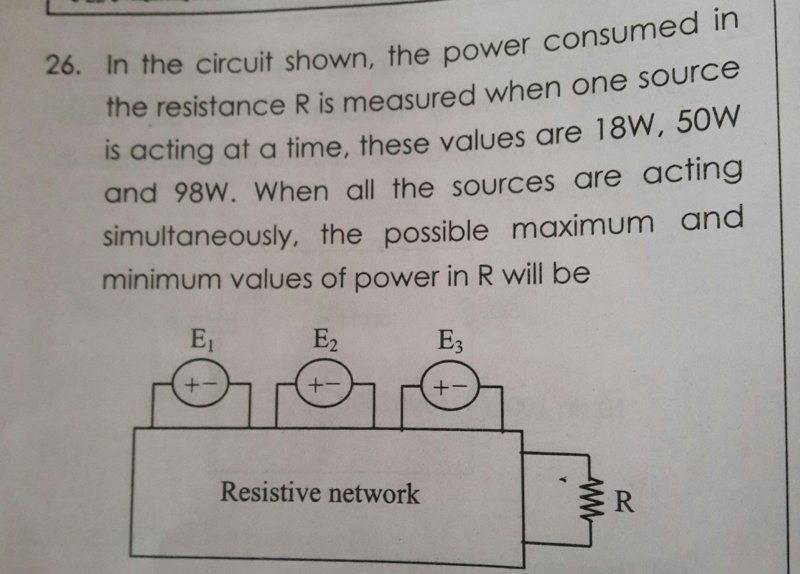# Electrical Network Problem: Power Transfer

Homework Helper
Gold Member

## Homework Statement## Homework Equations

P=V2/R
For maximum power transfer,

## The Attempt at a Solution

I know the efficiency is 50% during maximum power transfer. So when all the sources are acting simultaneously and ( at the same time) R=Rth, maximum power will be dissipated in R.
I am not sure how to use the given values of power since both R and the source voltages are unknown. Plus, the voltage sources are connected across three different ports. This problem is from Circuit Theorems chapter, so I guess I need to use Maximum power transfer theorem along with Superposition theorem.
Any guidance is appreciated.

#### Attachments

haruspex
Homework Helper
Gold Member
For maximum power transfer,
I don't think this is relevant here. That equation says how to adjust the load to get maximum power transfer into it.
Consider that the direction of current might change between the three given power results.

•cnh1995 and CWatters
Homework Helper
Gold Member
Consider that the direction of current might change between the three given power results.
Yes, but I don't know how to relate the three power results. I can't directly add/subtract the power results since I don't know the network inside the box. I think I need to set up an equation using some sort of proportionality (P∝V2 for instance).
The answer given is 450W (max) and 2W (min).
Do I need to use any specific network theorem here?

Homework Helper
Gold Member
Yes, but I don't know how to relate the three power results. I can't directly add/subtract the power results since I don't know the network inside the box. I think I need to set up an equation using some sort of proportionality (P∝V2 for instance).
The answer given is 450W (max) and 2W (min).
Do I need to use any specific network theorem here?
Plus, when they say 'one source is acting at a time', do I open-circuit the other two sources or short them?
I now doubt if this problem is solvable with the given data.

gneill
Mentor
Plus, when they say 'one source is acting at a time', do I open-circuit the other two sources or short them?
Presumably they mean to suppress the sources as is done in superposition analysis. So, short them since they are voltage sources.

Suppose that each individual source produces some potential difference across R. By superposition you know that these potential differences will sum linearly, and the resulting power will go as the square of that sum. But you are given individual power dissipations instead. How might you sum them?

•cnh1995
Homework Helper
Gold Member
Presumably they mean to suppress the sources as is done in superposition analysis. So, short them since they are voltage sources.

Suppose that each individual source produces some potential difference across R. By superposition you know that these potential differences will sum linearly, and the resulting power will go as the square of that sum. But you are given individual power dissipations instead. How might you sum them?
If E1, E2 and E3 produce voltages V1, V2 and V3 respectively across R individually, then in combined case,
P=V2/R,
where V=aV1+bV2+cV3.
Is that right?

gneill
Mentor
If E1, E2 and E3 produce voltages V1, V2 and V3 respectively across R individually, then in combined case,
P=V2/R,
where V=aV1+bV2+cV3.
Is that right?
Yes. You can also say that the individual potential differences expressed across R will be proportional to the square root of the individual powers.

•haruspex
Homework Helper
Gold Member
If E1, E2 and E3 produce voltages V1, V2 and V3 respectively across R individually, then in combined case,
P=V2/R,
where V=aV1+bV2+cV3.
Is that right?
That looks reasonable, but there are limits on a, b and c.

Homework Helper
Gold Member
As per post 7, given the termination has some ## R_o ##, ## P_a=\frac{V_a^2}{R_o} ##, ##P_b=\frac{V_b^2}{R_o} ##, and ## P_c=\frac{V_c^2}{R_o} ##. A little algebra gives ## \sqrt{P_a}+\sqrt{P_b}+\sqrt{P_c}=\frac{V_a+V_b+V_c}{\sqrt{R_o}}=\frac{V_{total}}{\sqrt{R_o}} ##. It is a simple matter to compute ## P_{total} ## by squaring both sides. ## \\ ## Edit: One additional item though: What if the sign of ## V_b ## or ## V_c ## is different from that of ## V_a ##? The above equality doesn't always hold. That's apparently why there is a maximum and a minimum. ## \\ ## Additional edit: And yes, I get the answers of post 3 by selecting the appropriate relative signs for the 3 terms.

Last edited:
•cnh1995
Homework Helper
Gold Member
Thanks again!•